Next: Worked example 6.5: Elastic Up: Conservation of momentum Previous: Worked example 6.3: Skater

## Worked example 6.4: Bullet and block

Question: A bullet of mass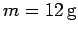strikes a stationary wooden block of mass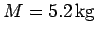standing on a frictionless surface. The block, with the bullet embedded in it, acquires a velocity of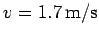. What was the velocity of the bullet before it struck the block? What fraction of the bullet's initial kinetic energy is lost (i.e., dissipated) due to the collision with the block?

Answer: Letbe the initial velocity of the bullet. Momentum conservation requires the total horizontal momentum of the system to be the same before and after the bullet strikes the block. The initial momentum of the system is simply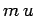, since the block is initially at rest. The final momentum is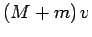, since both the block and the bullet end up moving with velocity. Hence,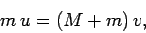giving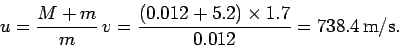The initial kinetic energy of the bullet is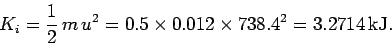The final kinetic energy of the system is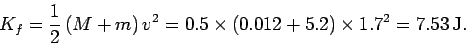Hence, the fraction of the initial kinetic energy which is dissipated is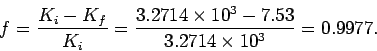Next: Worked example 6.5: Elastic Up: Conservation of momentum Previous: Worked example 6.3: Skater
Richard Fitzpatrick 2006-02-02